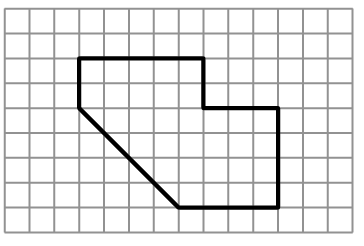### Home > CCG > Chapter 8 > Lesson 8.2.1 > Problem8-71

8-71.Examine the shape at right.

1. Find the area and perimeter of the shape.

Use the graph to find the lengths. Remember to draw in right triangles and use the Pythagorean Theorem to find the length.

$\text{Perimeter}\approx25.7 \; \text{units}$

2. On graph paper, enlarge the figure so that the linear scale factor is $3$. Find the area and perimeter of the new shape.

Multiply each of the dimensions of this figure by $3$ to draw the new, larger figure.

$\text{Perimeter}\approx77 \; \text{units}$

3. What is the ratio of the perimeters of both shapes? What is the ratio of the areas?

Remember that the ratio of the perimeters is not the same as the ratio of the areas.